• ×
السبت 6 يونيو 2020 | 05-28-2020
×

# Young's Double Slit Experiment تجربة يونج

In 1801 Thomas Young was able to offer some very strong evidence to support the wave model of light.

• He placed a screen that had two slits cut into it in front of a monochromatic (single color) light.
• The results of Young"s Double Slit Experiment should be very different if light is a wave or a particle.
• Let’s look at what the results would be in both situations, and then see how this experiment supports the wave model.

## If light is a particle…

We set up our screen and shine a bunch of monochromatic light onto it.

• If light is a particle, then only the couple of rays of light that hit exactly where the slits are will be able to pass through.Since they are little particles they will make a pattern of two exact lines on the viewing screen (Figure 1).
• Imagine it as being almost as though we are spraying paint from a spray can through the openings.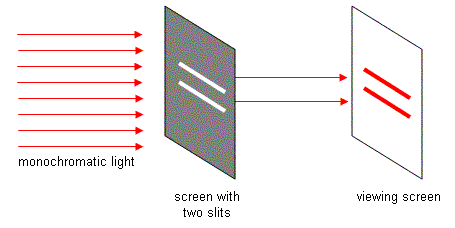Figure 1

## If light is a wave…

If light is a wave, everything starts the same way, but results we get are very different.

• There are still only two light rays that actually go through the slits, but as soon as they pass through they start to diffract.
• Remember, diffraction is when light passes through a small opening and starts to spread out. This will happen from both openings (Figure 2).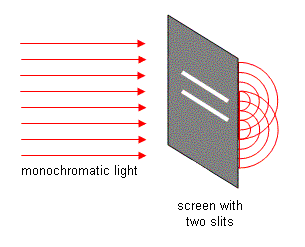Figure 2
• Notice that at some points the two sets of waves will meet crest to crest, at other spots crest meets trough.When this experiment is performed we actually see this, as shown in Figure 3.
• Where crest meets crest, there will be constructive interference and the waves will make it to the viewing screen as a bright spot.
• Where crest meets trough there will be destructive interference that cancel each other out… a black spot will appear on the screen.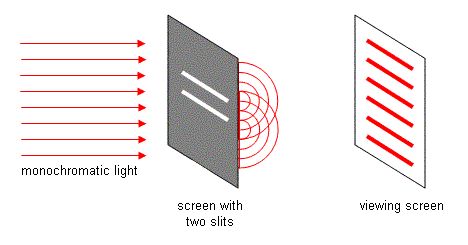Figure 3

We must conclude that light is made up of waves, since particles can not diffract.

## Calculations

When you set up this sort of an apparatus, there is actually a way for you to calculate where the bright lines (called fringes) will appear.

• There is always a middle line, which is the brightest. We call it the central fringe.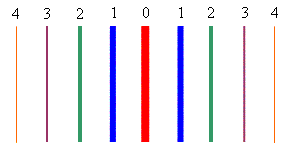Figure 4: Note I’ve only coloured the lines
differently here so that they will stand out
better. For monochromatic light they would
all have the same colour… that of the
original light.
• In the formula we will use, there is a variable, “n”, that is a count of how many bright fringes you are away from the central fringe.
• The central fringe is n = 0.
• The fringe to either side of the central fringe has an order of n = 1 (the first order fringe).
• The order of the next fringe out on either side is n = 2 (the second order fringe).
• And so on, as shown in Figure 4.

The formula that we will use to figure out problems involving double slit experiments is easy to mix up, so make sure you study it carefully.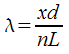λ = wavelength of light used (m)
x = distance from central fringe (m)
d = distance between the slits (m)
n = the order of the fringe
L = length from the screen with slits to the viewing screen (m)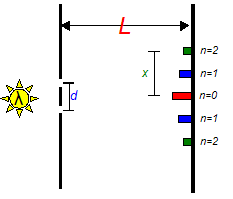Figure 5

It is very easy to mix up the measurements of x, d, and L.

• Make sure to look at Figure 5 and see the different things each is measuring.
• If you mix up x and d it"s not so bad, since they are both on top in the formula. If you were to mix them up with L, you would get the wrong answer.
• Almost all questions that you will see for this formula just involve sorting out what each variable is... you might find it helpful to write out a list of givens.

Example 1: A pair of screens are placed 13.7m apart. A third order fringe is seen on the screen 2.50cm from the central fringe. If the slits were cut 0.0960 cm apart, determinethe wavelength of this light. Roughly what colour is it?

Just to make sure you’ve got all the numbers from the question matched with the correct variables…

L = 13.7 m
n = 3
x = 2.50cm = 0.0250 m
d = 0.0960cm = 9.60e-4 m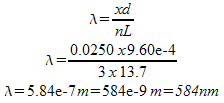It’s probably a yellow light being used given the wavelength we"ve measured.

If a white light is used in the double slit experiment, the different colours will be split up on the viewing screen according to their wavelengths.

• The violet end of the spectrum (with the shortest wavelengths) is closer to the central fringe, with the other colours being further away in order.

There is also a version of the formula where you measure the angle between the central fringe and whatever fringe you are measuring.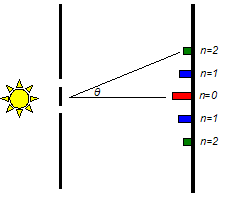Figure 6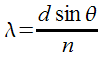• The formula works the same way, with the only difference being that we measure the angle instead of x and L.
• Make sure that your calculator is in degree mode before using this version of the formula.

Example 2: If a yellow light with a wavelength of 540 nm shines on a double slit with the slits cut 0.0100 mm apart, determine what angle you should look away from the central fringe to see the second order fringe?

Do not forget to:

1. Change the wavelength into metres.
2. Change the slit separation into metres.
3. "Second order" is a perfect number and has an infinite number of sig digs.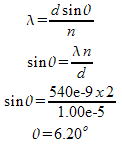## The Single Slit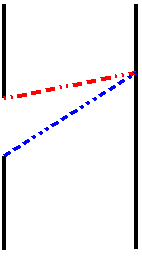Figure 7

A surprising experiment is that you can get the same effect from using a single slit instead of a double slit.

• Light from different ends of the slit will be traveling to the same spot on the screen and reach there either in or out of sync.If they meet crest to crest or trough to trough they will be in sync, but if they meet crest to trough they will be out of sync.
• In Figure 7, the blue path has to travel further than the red path... if this difference is equal to half a wavelength, they will meet each other out of sync.
• Being in sync will result in constructive interference, while meeting out of sync will result in destructive interference.
• The result is the same interference pattern, although the effect isn’t nearly as dramatic or clear as the double slit experiment.The only real difference in calculations is that "d" is now the width of the single opening.
• After the first couple of fringes (n = 1 and 2), the edges start getting really fuzzy, so you have a hard time measuring anything.
• If two slits work better than one, would more than two slits work better? This is a question that we will answer in the next section.

Example 3: For a single slit experiment apparatus like the one described above, determine how far from the central fringe the first order violet (λ = 350nm) and red (λ = 700nm) colours will appear if the screen is 10 m away and the slit is 0.050 cm wide.

We need to solve the formula for “x”, the distance from the central fringe.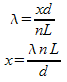For the violet light…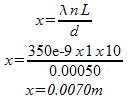For the red light…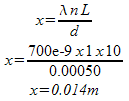بواسطة :
01-01-2017 12:00 صباحاً  0  0  2.0K
التعليقات ( 0 )